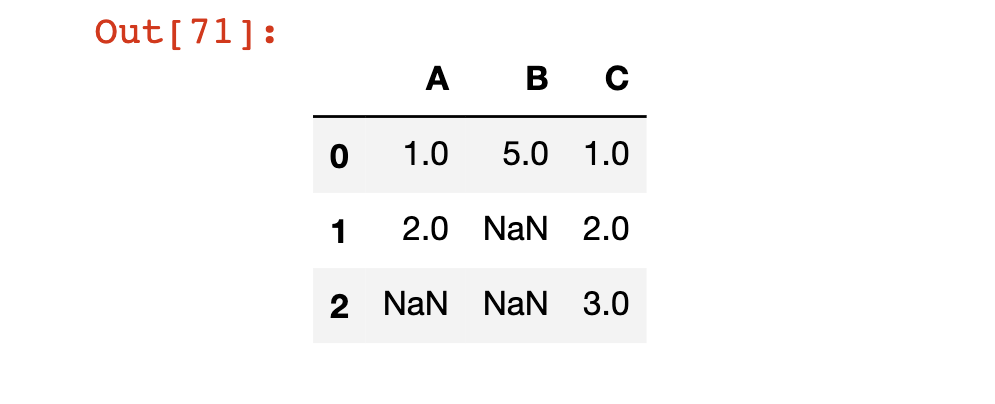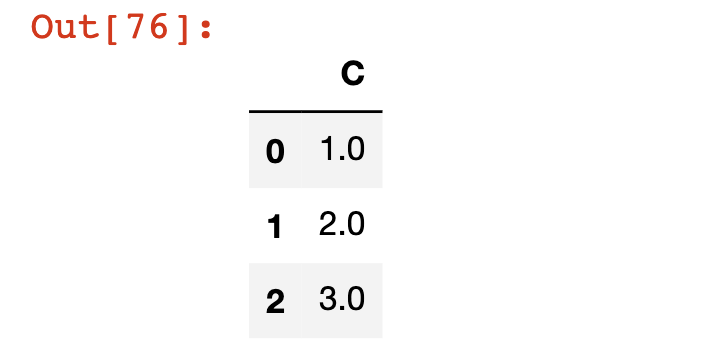# How To Deal With Missing Data in Pandas

Hey - Nick here! This page is a free excerpt from my \$199 course Python for Finance, which is 50% off for the next 50 students.

In an ideal world we will always work with perfect data sets. However, this is never the case in practice. There are many cases when working with quantitative data that you will need to drop or modify missing data. We will explore strategies for handling this throughout this lesson.

## The DataFrame We'll Be Using In This Lesson

We will be using the `np.nan` attribute to generate `NaN` values throughout this lesson.

``````Np.nan

#Returns nan``````

In this lesson, we will make use of the following DataFrame:

``````df = pd.DataFrame(np.array([[1, 5, 1],[2, np.nan, 2],[np.nan, np.nan, 3]]))

df.columns = ['A', 'B', 'C']

df``````## The Pandas `dropna` Method

Pandas has a built-in method called `dropna`. When applied against a DataFrame, the `dropna` method will remove any rows that contain a NaN value.

Let's apply the `dropna` method to our `df` DataFrame as an example:

``df.dropna()``Note that like the other DataFrame operations that we have explored, `dropna` does not modify the original DataFrame unless you either (1) force it to using the `=` assignment operator or (2) specify `inplace=True`.

We can also drop any columns that have missing values by passing in the `axis=1` argument to the `dropna` method, like this:

``df.dropna(axis=1)``## The Pandas `fillna` Method

In many cases, you will want to replace missing values in a pandas DataFrame instead of dropping it completely. The `fillna` method is designed for this.

As an example, let's fill every missing value in our DataFrame with the `🔥`:

``df.fillna('🔥')``Obviously, there is basically no situation where we would want to replace missing data with an emoji. This was simply an amusing example.

Instead, more commonly we will replace a missing value with either:

• The average value of the entire DataFrame
• The average value of that row of the DataFrame

We will demonstrate both below.

To fill missing values with the average value across the entire DataFrame, use the following code:

``df.fillna(df.mean())``

To fill the missing values within a particular column with the average value from that column, use the following code (this is for column `A`):

``df['A'].fillna(df['A'].mean())``

## Moving On

In this lesson we explored the `dropna` and `fillna` methods for dealing with missing data in pandas. After working through some practice problems, we will discuss how to group a DataFrame's elements according to a certain characteristic next.